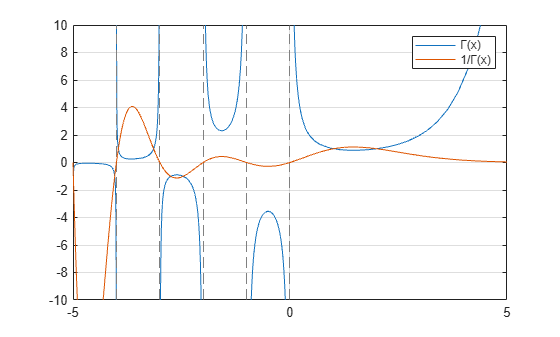# gamma

Gamma function

## Syntax

``Y = gamma(X)``

## Description

example

````Y = gamma(X)` returns the `gamma` function evaluated at the elements of `X`. ```

## Examples

collapse all

Evaluate the gamma function with a scalar and a vector.

Evaluate $\Gamma \left(0.5\right)$, which is equal to $\sqrt{\pi }$.

`y = gamma(0.5)`
```y = 1.7725 ```

Evaluate several values of the gamma function between `[-3.5 3.5]`.

```x = -3.5:3.5; y = gamma(x)```
```y = 1×8 0.2701 -0.9453 2.3633 -3.5449 1.7725 0.8862 1.3293 3.3234 ```

Plot the gamma function and its reciprocal.

Use `fplot` to plot the gamma function and its reciprocal. The gamma function increases quickly for positive arguments and has simple poles at all negative integer arguments (as well as 0). The function does not have any zeros. Conversely, the reciprocal gamma function has zeros at all negative integer arguments (as well as 0).

```fplot(@gamma) hold on fplot(@(x) 1./gamma(x)) ylim([-10 10]) legend('\Gamma(x)','1/\Gamma(x)') hold off grid on```## Input Arguments

collapse all

Input array, specified as a scalar, vector, matrix, or multidimensional array. The elements of `X` must be real.

Data Types: `single` | `double`

## Limitations

• For `double` and `single` data types, the `gamma` function returns `Inf` for all values greater than `realmax` and `realmax('single')`. The saturation thresholds for positive integers are `gamma(172)` and `gamma(single(36))`, where the evaluated `gamma` functions are greater than the maximum representable values.

collapse all

### Gamma Function

The `gamma` function is defined for real `x > 0` by the integral:

`$\Gamma \left(x\right)={\int }_{0}^{\infty }{e}^{-t}{t}^{x-1}dt$`

The `gamma` function interpolates the `factorial` function. For integer `n`:

`gamma(n+1) = factorial(n) = prod(1:n)`

The domain of the `gamma` function extends to negative real numbers by analytic continuation, with simple poles at the negative integers. This extension arises from repeated application of the recursion relation

`$\Gamma \left(n-1\right)=\frac{\Gamma \left(n\right)}{n-1}\text{\hspace{0.17em}}.$`

## Algorithms

The computation of `gamma` is based on algorithms outlined in .

 Cody, J., An Overview of Software Development for Special Functions, Lecture Notes in Mathematics, 506, Numerical Analysis Dundee, G. A. Watson (ed.), Springer Verlag, Berlin, 1976.

 Abramowitz, M. and I.A. Stegun, Handbook of Mathematical Functions, National Bureau of Standards, Applied Math. Series #55, Dover Publications, 1965, sec. 6.5.